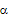Problem 76. Area of a Circle, Square,  Circular Sector, Lune. Level: High School, College, SAT Prep. Given a square ABCD 1, the inscribed circle 2, the arc BD of center A. S is the area of the lune EF, S1, S2, and S3 are the shaded areas. Prove that S = S1 + S2 + S3. See also: Artwork Problem 76.HINTS: AREA OF A CIRCLE. The area enclosed by a circle is π multiplied by the radius squared.CIRCULAR SECTOR: or a sector of a circle is a part of a circle bounded by two radii and their intercepted arc. AREA OF A CIRCULAR SECTOR: In a circle of radius r, the area A of a sector of angle(degrees) equalsof the area of the circle:Recent Additions